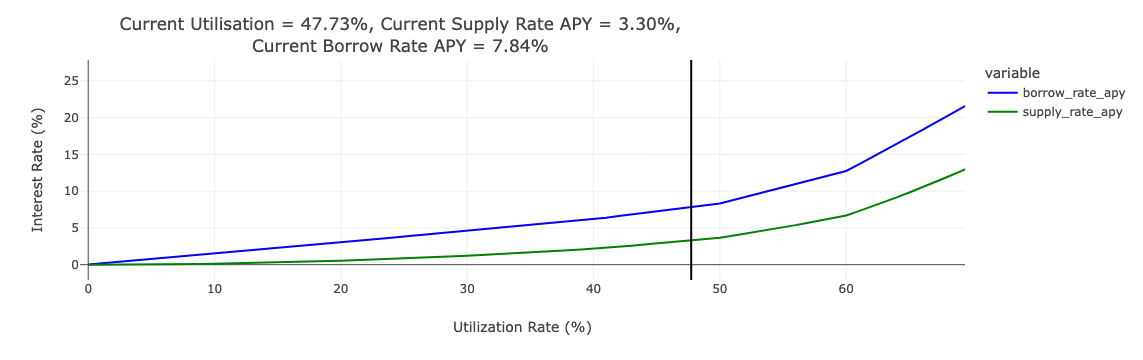Comment on page

# Interest Rates

Interest rates are dictated by the utilization (
$U$
), of an asset. When
$U$
is low, it means there is excess supply and low borrow demand. As
$U$
moves closer to 100%, supply and borrow rates will increase, thus:
• Encouraging lenders to supply into the market
• Encouraging borrowers to repay debt
To mitigate the risk of a token reserve reaching 100% utilization, K-Lend employs a Poly-linear interest rate curve.
This approach balances the dual objectives of maximizing interest earned by lenders while effectively managing liquidity risk. The parameters of the interest rate curve are calibrated by the Risk Council to align with a predetermined target utilization rate.

### Borrow Interest Rate

The current borrowing rate,
$B\displaystyle_t$
, is determined by the current utilization rate,
$U\displaystyle_t$
, and the discrete function
$f(U\displaystyle_i)\rightarrow B\displaystyle_i$
mapping utilization to borrow rate as specified knot points.
$B\displaystyle_t = B\displaystyle_F + \frac{B\displaystyle_C -B\displaystyle_F}{U\displaystyle_C -U\displaystyle_F}(U\displaystyle_t -U\displaystyle_F)$
Where:
$B\displaystyle_t$
:= Current borrow interest rate
$B\displaystyle_C$
:= Borrow interest rate at the ceiling utilization knot point
$B\displaystyle_F$
:= Borrow interest rate at the floor utilization knot point
$U\displaystyle_t$
:= Current utilization rate
$U\displaystyle_C$
:= Utilization rate at the ceiling knot point
$U\displaystyle_F$
:= Utilization rate at the floor knot point

### Supply Interest Rate

Due to the fact that K-Lend has only variable rate borrows, as opposed to stable borrow rates, the Supply Rate equation is quite simple:
$S\displaystyle_t = U\displaystyle_t * B\displaystyle_t(1 -R\displaystyle_t)$
Where:
$S\displaystyle_t$
:= Current supply interest rate
$B\displaystyle_t$
:= Current borrow interest rate
$R\displaystyle_t$
:= Current Reserve Factor (Protocol take rate)

### Curve ExampleInterest Rate Curve Example